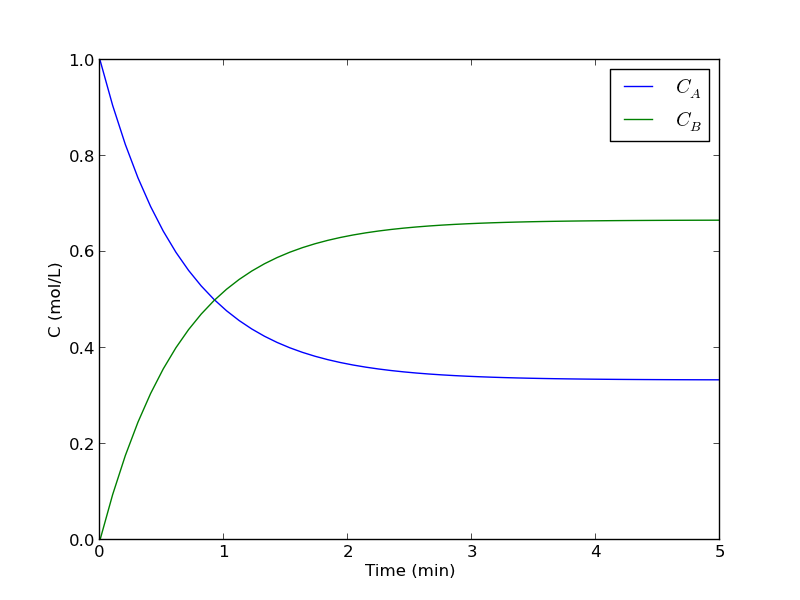## Time dependent concentration in a first order reversible reaction in a batch reactor

| categories: ode | tags: reaction engineering

Given this reaction $$A \rightleftharpoons B$$, with these rate laws:

forward rate law: $$-r_a = k_1 C_A$$

backward rate law: $$-r_b = k_{-1} C_B$$

plot the concentration of A vs. time. This example illustrates a set of coupled first order ODES.

from scipy.integrate import odeint
import numpy as np

def myode(C, t):
# ra = -k1*Ca
# rb = -k_1*Cb
# net rate for production of A:  ra - rb
# net rate for production of B: -ra + rb

k1 = 1   # 1/min;
k_1 = 0.5   # 1/min;

Ca = C
Cb = C

ra = -k1 * Ca
rb = -k_1 * Cb

dCbdt = -ra + rb

return dCdt

tspan = np.linspace(0, 5)

init = [1, 0]  # mol/L
C = odeint(myode, init, tspan)

Ca = C[:,0]
Cb = C[:,1]

import matplotlib.pyplot as plt
plt.plot(tspan, Ca, tspan, Cb)
plt.xlabel('Time (min)')
plt.ylabel('C (mol/L)')
plt.legend(['$C_A$', '$C_B$'])
plt.savefig('images/reversible-batch.png')That is it. The main difference between this and Matlab is the order of arguments in odeint is different, and the ode function has differently ordered arguments.